# EnzymeKinetics

Enzyme Kinetics. (work in progress, feel free to add/edit/correct!)

Consider the following enzymatic reaction (E is the enzyme, S the substrate, ES an enzyme substrate complex and P the product).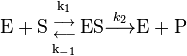${\mathrm{E}} + {\mathrm{S}} {{{\mathrm{k_1}}\atop{\longrightarrow}} \atop{{\longleftarrow}\atop{{\mathrm{k_{-1}}}}}} {\mathrm{ES}} \stackrel{k_2}{\longrightarrow} \mathrm{E} + \mathrm{P}$

The Michaelis Menten equation is often used to calculate the rate of enzymatic reactions and is arrived at using either the pseudo steady state (the rate of change of ES with time is small, almost zero) or the equilibrium assumption (that ES is always in equilibrium with E and S, the first step in the reaction). We show how this is arrived at and how to solve the equation.

The elementary reactions are: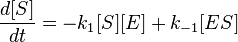$\frac{d[S]}{dt} = -k_1 [S][E] + k_{-1}[ES]$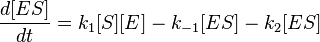$\frac{d[ES]}{dt} = k_1[S][E] - k_{-1}[ES] - k_2[ES]$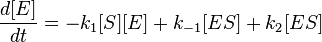$\frac{d[E]}{dt} = -k_1 [S][E] + k_{-1}[ES] + k_2[ES]$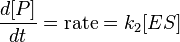$\frac{d[P]}{dt} = \mathrm{rate} = k_2 [ES]$

There are two ways to solve for [E'S] so we can get a rate expression that includes the substrate concentration and parameters that can characterize the nature of the enzyme.

The pseudo steady state (PSS) approximation states that we can assume that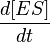$\frac{d[ES]}{dt}$ is zero, while the equilibrium assumption states that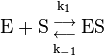${\mathrm{E}} + {\mathrm{S}} {{{\mathrm{k_1}}\atop{\longrightarrow}} \atop{{\longleftarrow}\atop{{\mathrm{k_{-1}}}}}} {\mathrm{ES}}$ is at equilibrium. Either of these assumptions/approximations will lead you to the Michaelis Menten form of the rate expression, written as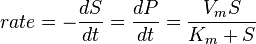$rate = -\frac{dS}{dt} = \frac{dP}{dt} = \frac{V_m S}{K_m + S}$ where Vm can be shown to equal k2E0 where

E0 is the initial concentration of the enzyme Km is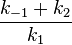$\frac{k_{-1}+k_2}{k_1}$. (If you use the equilibrium assumption, you get a very similar expression, where the Km is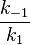$\frac{k_{-1}}{k_1}$ (PSS is considered a better approximation to use)

The PSS approximation is a good one to make, as long as the substrate concentration is much larger than that of the enzyme (you can check this by manipulating these concentrations in the model below that includes the four differential equations, see what happens if substrate is NOT much larger than Enzyme concentration, the PSS assumption breaks down)

In the PyGTK GUI, you can plot any of the dependent variable (Substrate (S), Enzyme (E), EnzymeSubstrate Complex (ES) or product (P)) as a function of the independent variable (time)

### The Michaelis Menten Equation (Simplified Form)

REQUIRE "ivpsystem.a4l";
REQUIRE "atoms.a4l";

MODEL lowKm;
dS_dt IS_A rate;
Vm IS_A rate;

Km, S IS_A factor;
dS_dt = -Vm*S/(Km + S);

t IS_A time;
METHODS
RUN default_self;

RUN reset; RUN values;
RUN set_obs;

RUN set_ode;

METHOD default_self;

END default_self;
METHOD specify;
FIX Km, Vm, S;

END specify;
METHOD values;
S := 10.0;

dS_dt := 0 {Hz};
Vm  := 1.0 {1/s};

Km := 20.0;
t   := 0{s};
END values;

METHOD set_obs;
S.obs_id :=1;
END set_obs;

METHOD setup;
RUN specify;
RUN values;
END setup;

METHOD set_ode;
FREE dS_dt;
S.ode_id := 1;   dS_dt.ode_id := 1;

dS_dt.ode_type := 2;
S.ode_type := 1;

t.ode_type :=-1;

END set_ode;
END lowKm;

MODEL highKm;

dS_dt IS_A rate;
Vm IS_A rate;

Km, S IS_A factor;
dS_dt = -Vm*S/(Km + S);

t IS_A time;

METHODS

RUN default_self;
RUN reset; RUN values;

RUN set_obs;
RUN set_ode;

METHOD default_self;
END default_self;

METHOD specify;

FIX Km, Vm, S;
END specify;

METHOD values;
S := 10.0;
dS_dt := 0 {Hz};

Vm  := 1.0 {1/s};
Km := 200.0;
t   := 0{s};

END values;
METHOD set_obs;
S.obs_id :=1;

END set_obs;

METHOD setup;
RUN specify;
RUN values;

END setup;

METHOD set_ode;
FREE dS_dt;

S.ode_id := 1;   dS_dt.ode_id := 1;

dS_dt.ode_type := 2;
S.ode_type := 1;

t.ode_type :=-1;

END set_ode;

END highKm;


### Solving the Set of Ordinary Differential Equations

REQUIRE "ivpsystem.a4l";

REQUIRE "atoms.a4l";

MODEL simple;

dES_dt IS_A rate;
dS_dt IS_A rate;

dP_dt IS_A rate;
dE_dt IS_A rate;
k1, k2, km1 IS_A rate;

P, E, S, ES IS_A positive_factor;

eq1: dES_dt = k1*E*S - km1*ES - k2*ES;

eq2: dS_dt = -k1*E*S + km1*ES;

eq3: dP_dt = k2*ES;
eq4: dE_dt = -k1*E*S + km1*ES + k2*ES;

t IS_A time;

METHODS

RUN default_self;
RUN specify;

RUN values;

RUN set_obs;

RUN set_ode;

METHOD default_self;
END default_self;

METHOD specify;

FIX k1, km1, k2;
FREE dS_dt, dP_dt, dE_dt, dES_dt;

FREE S, E, P, ES;
FIX E, S, P, ES;

END specify;

METHOD values;
ES := 0;

P := 0;
S := 1.0;
E := 0.1;

dS_dt := 0 {Hz};
dES_dt := 0 {Hz};

dP_dt := 0 {Hz};
dE_dt := 0 {Hz};

k1 := 1.0 {1/s};
km1 := 0.02 {1/s};

k2 := 0.02 {1/s};
t   := 0 {s};

END values;

METHOD set_obs;
ES.obs_id :=1;

E.obs_id := 4;
S.obs_id := 2;

P.obs_id := 3;
END set_obs;

METHOD set_ode;

S.ode_id := 1;   dS_dt.ode_id := 1;

ES.ode_id := 2; dES_dt.ode_id := 2;

E.ode_id := 3; dE_dt.ode_id := 3;

P.ode_id := 4; dP_dt.ode_id := 4;

dS_dt.ode_type := 2; dES_dt.ode_type := 2; dP_dt.ode_type := 2; dE_dt.ode_type := 2;

S.ode_type := 1; E.ode_type := 1; ES.ode_type := 1; P.ode_type := 1;

t.ode_type :=-1;

END set_ode;
END simple;